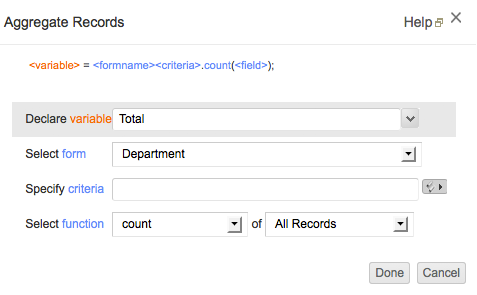# Aggregate Records

## Overview

The Aggregate Records task supports for calculating SUM/COUNT/AVG/MIN/MAX of a Form's field values. This will help creating Applications without iterating the records.
Example usage julymonthsales = Order_Info[Month == 7].sum(amount);

• Count: Returns the count of all or specific records in a form.
• Sum: Returns the sum of a numeric field for all or selected records in the form
• Average: Returns the average of a numeric field for all or selected records in the form
• Maximum: Returns the maximum value of a numeric /date-time field, from all or selected records in the form
• Minimum: Returns the minimum value of a numeric /date-time field, from all or selected records in the form
• Distinct: Returns the distinct values of a field in a form.

## Syntax

### Count Function

//to count all records in a form

//to count specific records with a given criteria
<variable> = <Form_LinkName>[criteria expression].count();

where,
<variable> refers to a user defined variable name or a form field.

#### Example 1

//to count all records in a form
input.Total = FormA.count();

//to count specific records with a given criteria
input.Food_Expenses = FormA[Expense == "Food"].count();

where FormA is the Form Link Name

#### Example 2

Lets say you have a form with a date field. To fetch records added on a specific date, add the following code to the required form actions block:

Day_Count = Form[Date_field = "07-Sep-2014"].count()

where,
Day_count is the variable that holds the count of records on 07-Sep-2014.
Form is the form link name .
Date_field = "07-Sep-2014" is the criteria, where Date_field is the date field in the Form.
You can replace the date value with input.Date_field to select the date value entered in the form.

### Sum Function

//to sum the total value of a numeric field in the form
<variable> = <Form_Name>.sum(<Numeric Field Name>);

//to sum specific records with a given criteria
<variable> = <Form_Name>[criteria expression].sum(<Numeric Field Name>);

where,
<variable> refers to a user defined variable name or a form field.

Example:

//to find total sales value
Total_Sales = FormA.sum(Amount);

//to find the total sales value for Item="Printer"
ItemA = FormA[Item == "Printer"].sum(Amount);

### Average Function

//to find the average of a numeric field, for all records
<variable> = <Form_Name>.avg(<Numeric Field Name>);

//to find the average of a numeric field for specific records
<variable> = <Form_Name>[criteria expression].avg(<Numeric Field Name>);

where,
<variable> refers to a user defined variable name or a form field.

Example:

//to find average sales for all records
Avg_Sales = FormA.avg(Amount);

//to find the average sales for item="Printer"
Avg_Sales_ItemA = FormA[Item == "Printer"].avg(Amount);

### Maximum Function

//to find the maximum value of a numeric field, for all records
<variable> = <Form_Name>.maximum(<Numeric Field Name>);

//to find the maximum value of a numeric field for specific records
<variable> = <Form_Name>[criteria expression].maximum(<Numeric Field Name>);

where,
<variable> refers to a user defined variable name or a form field.

Example:

//to find the highest sale value, from all records
Highest_Sale_Val = FormA.maximum(Amount);

//to find the highest sale value for "Printer"
Highest_Sale_Val_ItemA = FormA[Item == "Printer"].maximum(Amount);

### Minimum Function

//to find the minimum value of a numeric field, for all records
<variable> = <Form_Name>.minimum(<Numeric Field Name>);

//to find the mimimum value of a numeric field for specific records
<variable> = <Form_Name>[criteria expression].minimum(<Numeric Field Name>);

where,
<variable> refers to a user defined variable name or a form field.

Example:

//to find the lowest sale value, from all records
Highest_Sale_Val = FormA.minimum(Amount);

//to find the lowest sale value for "Printer"
Highest_Sale_Val_ItemA = FormA[Item == "Printer"].minimum(Amount);

### Distinct Function

//to get distinct fields values from a form <list-variable> = <Form_Name>.distinct(<fieldname>);
//to get distinct values from a form with a given criteria <list-variable> = <Form_Name>[criteria expression].distinct(<fieldname>);

where,

<list-variable> refers to a user defined list variable name or a form field.

## Using Script Builder

The Aggregate Records task can be configured from the Script builder as specified in the steps given below:

1. Drag-and-drop the aggregate records task from the left-side (as highlighted in the screen-shot below). Click on the Edit button to configure the values in the Aggregate records dialog.
2. Declare a variable or select a field name as variable to store the value returned by the Aggregate Records task.
3. Select the form from the list of forms displayed in the Select Form list.
4. Specify the required criteria. If no criteria is specified the aggregate function will be executed on all records.
5. Select the aggregate function from the list and the field name on which the function will be executed.
6. Click Done and Save Script.